# Stage 2

### Member Training: Group Comparisons

August 31st, 2023 byHow do you know which method to use when you want to compare groups?
(more…)

### Member Training: The Link Between ANOVA and Regression

January 31st, 2023 byIf you’ve used much analysis of variance (ANOVA), you’ve probably heard that ANOVA is a special case of linear regression. Unless you’ve seen why, though, that may not make a lot of sense. After all, ANOVA compares means between categories, while regression predicts outcomes with numeric variables.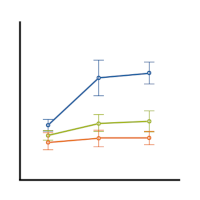(more…)

### When the Results of Your ANOVA Table and Regression Coefficients Disagree

December 8th, 2022 by

Have you ever had this happen? You run a regression model. It can be any kind—linear, logistic, multilevel, etc. In the ANOVA table, the effect of interest has a very low p-value. In the regression table, it doesn’t. Or vice-versa.

How can the same effect have two different p-values? In this article, let’s explore when this happens and what it means.

### What the statistics in each table measures

The ANOVA table is a table of F tests. It may not be called the ANOVA table on your output, but it always includes a set of F tests. Some software procedures only give one F test for the model as a whole, but most will break it down into a series of F tests, one for each predictor variable or term in your model.

The regression coefficients table is a table of t tests. It includes each regression coefficient, along with its standard error, and usually a t test (some generalized linear models will have Wald or z tests instead, but they have the same role here).

Both tables often list out each predictor variable, along with a p-value for that variable’s conditional effect on Y.

There are two situations in which the p-values will match. Both must be true.

1. The F test has one df. This happens in two situations. Either the predictor, X, is numerical or it’s categorical and binary (only two groups).
2. The predictor is not involved with any interactions with a variable that is not centered at is mean.

If both of those are true, not only will the p-value match, but the t-statistic in the regression coefficients table will be the positive or negative square root of the F statistic.

### An Example ANOVA Table with Matching and Unmatching Regression Coefficients

Here’s an example of an ANOVA table from a linear regression. In this example, there are four treatment groups, two genders, and age in years (measured continuously and centered at its mean). The response variable, Y, is a satisfaction score with a training. The four groups represented four learning strategies the adult learners were trained to use.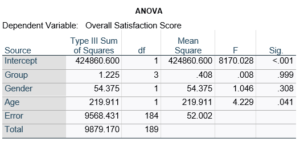Let’s compare this to the regression coefficients table.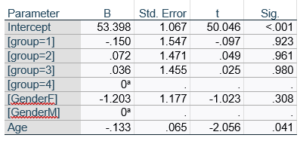If you compare p-values across the two tables, you can see that Gender and Age have the same p-values, but Group doesn’t.

Gender and Age meet both conditions. Both have 1 df in the F table. Gender because it’s binary (two categories) and Age because it’s numerical). There are no interactions.

Group doesn’t match because it has 3 df in the F test. The F test is testing the null hypothesis that there is no difference among the four means. The t-tests in the regression coefficients table are testing three specific contrasts. Each one compares one group mean to the group 4 mean. For example, the group=1 coefficient tests whether the difference between the mean group 1 satisfaction score differs only from the group 4 score. It’s a different null hypothesis than the F test.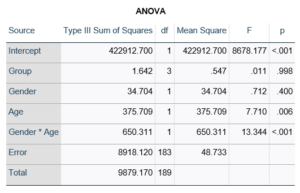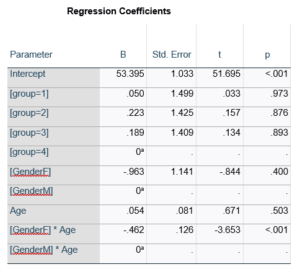This would be the case whether or not there were interactions in the model that contain Group. Any time you have more that one df in the F test (you can see group has 3), you’ll get as many p-values in the regression coefficients as you have df in the F table. The p-values can’t match because there are more of them in the regression coefficients table.

Gender, which is also categorical, does have the same p-value in both tables. It has 1 df in the F test, which tests the null hypothesis that the two gender means have no variance (they’re the same). Gender is involved in an interaction, so the only reason the hypothesis test, and therefore the p-value, is the same is because the variable it interacts with, Age, is centered.

In conclusion, most of the time, it’s fine if the results don’t match. It’s because the two tables are reporting results of different hypothesis tests, based on what’s in your model.

### Member Training: Centering

November 30th, 2022 byCentering variables is common practice in some areas, and rarely seen in others. That being the case, it isn’t always clear what are the reasons for centering variables.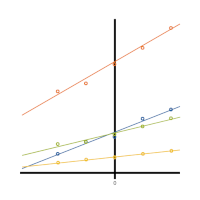Is it only a matter of preference, or does centering variables help with analysis and interpretation? (more…)

### What is a Completely Randomized Design?

November 8th, 2022 byThe most basic experimental design is the completely randomized design. It is simple and straightforward when plenty of unrelated subjects are available for an experiment. It’s so simple, it almost seems obvious. But there are important principles in this simple design that are important for tackling more complex experimental designs.

Let’s take a look.

### How It Works

The basic idea of any experiment is to learn how different conditions or versions of a treatment affect an outcome. To do this, you assign subjects to different treatment groups. You then run the experiment and record the results for each subject.

Afterward, you use statistical methods to determine whether the different treatment groups have different outcomes.

Key principles for any experimental design are randomization, replication, and reduction of variance. Randomization means assigning the subjects to the different groups in a random way.

Replication means ensuring there are multiple subjects in each group.

Reduction of variance refers to removing or accounting for systematic differences among subjects. Completely randomized designs address the first two principles in a simple way.

To execute a completely randomized design, first determine how many versions of the treatment there are. Next determine how many subjects are available. Divide the number of subjects by the number of treatments to get the number of subjects in each group.

The final design step is to randomly assign individual subjects to fill the spots in each group.

### Example

Suppose you are running an experiment. You want to compare three training regimens that may affect the time it takes to run one mile. You also have 12 human subjects who are willing to participate in the experiment. Because you have three training regimens, you will have 12/3 = 4 subjects in each group.

Statistical software (or even Excel) can do the actual assignment. You only need to start by numbering the subjects from 1 to 12 in any way that is convenient. The following table shows one possible random assignment of 12 subjects to three groups.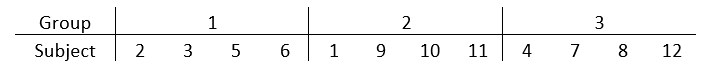It’s okay if the number of replicates in each group isn’t exactly the same. Make them as even as possible and assign more to groups that are more interesting to you. Modern statistical software has no trouble adjusting for different sample sizes.

When there is more than one treatment variable, not much changes. Use the combination of treatments when performing random assignment.

For example, say that you add a diet treatment with two conditions in addition to the training. Combined with the three versions of training, there are six possible treatment groups. Assign the subjects in the exact way already described, but with six groups instead of three.

Do not skip randomization! Randomization is the only way to ensure your groups are similar except for the treatment. This is important to ensuring you can attribute group differences to the treatment.

### When This Design DOESN’T Work

The completely randomized design is excellent when plenty of unrelated subjects are available to sample.  But some situations call for more advanced designs.

This design doesn’t address the third principle of experimental design, reduction of variance.

Sure, you may be able to address this by adding covariates to the analysis. These are variables that are not experimentally assigned but you can measure them. But if reduction of variance is important, other designs do this better.

If some of the subjects are related to each other or a single subject is exposed to multiple conditions of a treatment, you’re going to need another design.

Sometimes it is important to measure outcomes more than once during experimental treatment. For example, you might want to know how quickly the subjects make progress in their training. Again, any repeated measures of outcomes constitute a more complicated design.

### Strengths of the Completely Randomized Design

When it works, it has many strengths.

It’s not only easy to create, it’s straightforward to analyze. The results are relatively easy to explain to a non-statistical audience.

Finally, familiarity with this design will help you recognize when it isn’t appropriate. Understanding the ways in which it is not appropriate can help you choose a more advanced design.

### The Difference Between R-squared and Adjusted R-squared

August 22nd, 2022 by

R², the the Coefficient of Determination, is one of the most useful and intuitive statistics we have in linear regression.